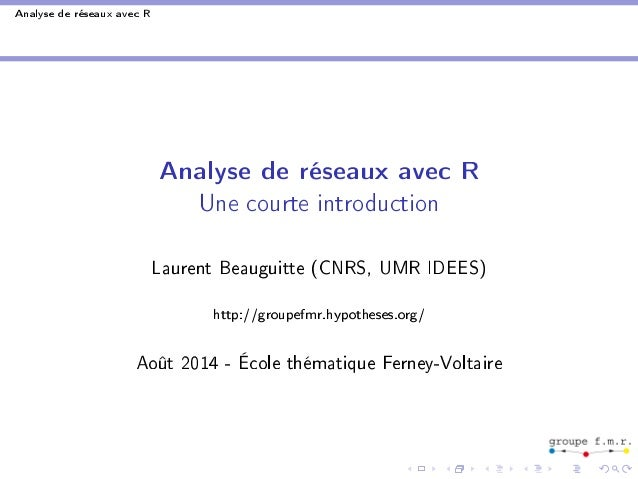Successfully reported this slideshow.
We use your LinkedIn profile and activity data to personalize ads and to show you more relevant ads. You can change your ad preferences anytime.Upcoming SlideShare
×

# Introduction à l'analyse de réseaux avec R

4,331 views

Published on

Présentation faite à l'école d'été Ferney-Voltaire 2014 (http://ferney2014.sciencesconf.org/) : initiation à l'analyse de réseaux avec R (packages statnet et igraph)

Published in: Software
• Full Name
Comment goes here.

Are you sure you want to Yes No
Your message goes hereAre you sure you want to  Yes  No
Your message goes here

### Introduction à l'analyse de réseaux avec R

1. 1. Analyse de réseaux avec R Analyse de réseaux avec R Une courte introduction Laurent Beauguitte (CNRS, UMR IDEES) http://groupefmr.hypotheses.org/ Août 2014 - École thématique Ferney-Voltaire
2. 2. Analyse de réseaux avec R Questions & principes de base I Pourquoi R ? I Interface de RStudio I Principes généraux I Obtenir de l'aide. . . I Créer des objets I Importer des chiers (read.table) I Contrôler l'import (str)
3. 3. Analyse de réseaux avec R Deux packages généralistes (statnet et igraph) non compatibles : utiliser l'un ou l'autre Pour démarrer une session : rm(list=ls()) # vider l'espace de travail library(statnet) # charger le package ou rm(list=ls()) # vider l'espace de travail library(igraph) # charger le package
4. 4. Analyse de réseaux avec R Visualiser un jeu de données rm(list=ls()) library(statnet) gplot(rgraph(10, tprob=0.2)) #random graph, 10 sommets #proba 20% library(igraph) plot(erdos.renyi.game(10, 1/5))
5. 5. Analyse de réseaux avec R Mesures de base statnet igraph densité gden graph.density comp. connexes components clusters degré degree degree betweenness betweenness betweenness closeness closeness closeness PCC* geodist shortest.paths cliques clique.census cliques k-cores kcores graph.coreness *PCC : plus court chemin
6. 6. Analyse de réseaux avec R Saisir les données : éditeur de texte (Notepad++), format .txt ou .csv Importer des données #liste de liens : origine, destination, attributs library(statnet) d - read.table(data.csv, header=TRUE, sep=;) g - as.network(as.matrix(d)) g library(igraph) g - graph.data.frame(d) g
7. 7. Analyse de réseaux avec R Importer des attributs pour les sommets (avec igraph) Une liste de liens origine - destination - attribut 1 - attribut 2, etc. Une liste de sommets - attribut 1 - attribut 2, etc rm(list=ls()) liens - read.table(liens.csv, header=TRUE, sep=;) g - graph.data.frame(liens) sommets - read.table(sommets.csv, header=TRUE, sep=;) V(g)\$Att=sommets\$A[match(V(g)\$name,sommets\$S)] # S = code des sommets, A = attribut des sommes #variation taille selon attribut plot(g, vertex.size = as.numeric(V(g)\$Att)*10)
8. 8. Analyse de réseaux avec R Idem avec statnet rm(list=ls()) library(statnet) d - read.table(liens.csv, header=TRUE, sep=;) str(d) da - read.table(sommets.csv, header=TRUE, sep=;) str(d) g - as.network(as.matrix(d[,-3])) g g %v% AGE - da\$A gplot(g, gmode=graph, displaylabels=TRUE, vertex.cex= da\$A)
9. 9. Analyse de réseaux avec R Graphe biparti (pas de mesure possible - statnet - ou résultats faux - igraph) rm(list=ls()) library(igraph) d - read.table(biparti.csv, sep=;, header=FALSE) g - graph.data.frame(d, directed = FALSE) g library(statnet) g - as.network(as.matrix(d), bipartite = TRUE) g
10. 10. Analyse de réseaux avec R Extraire et visualiser les ego-networks library(statnet) ego - ego.extract(g) gplot(ego\$a, displaylabels=TRUE, labels=colnames(ego\$a), gmode=graph)
11. 11. Analyse de réseaux avec R Soit le graphe suivant : I quel est le diamètre du graphe ? I combien y-a-t'il de cliques avec 3 sommets ? avec 4 ? I quels sont les sommets les plus centraux ? en terme de degré ? en terme d'intermédiarité ?
12. 12. Exercice 1 I créer le chier de liens correspondant à cette gure (format conseillé .csv) ; I l'importer dans R ; I le transformer en objet igraph ou network ; I chercher les cliques ; I mesurer le diamètre, le degré, l'intermédiarité.
13. 13. Analyse de réseaux avec R Avec statnet et/ou igraph, visualiser en faisant apparaître les attributs des sommets (couleur), le degré (taille) et l'intensité des liens (épaisseur). Exemples statnet igraph
14. 14. Analyse de réseaux avec R Structure des données exoliens.csv exosommets.csv O;D;I code;attribut a;b;3 a;1 a;c;2 b;1 a;d;1 c;1 ... d;2 ... Si vous changez les noms de colonnes, il faudra adapter le code donné ensuite Et il y aura la solution ensuite. . .
15. 15. rm(list=ls()) library(igraph) #import des fichiers liens et sommets lien - read.table(exoliens.csv, sep=;, header=TRUE) somm - read.table(exosommets.csv, sep=;, header=TRUE) #transformation en objet igraph #import de l'attribut des sommets g - graph.data.frame(lien, directed= FALSE) V(g)\$Att -somm\$attribut[match(V(g)\$name,somm\$code)] #mesures graph.density(g) degree(g) betweenness(g) max(shortest.paths(g)) cliques(g, min = 3)
16. 16. #visualisation colors = c(Red,Yellow) V(g)\$Att[V(g)\$Att == 1] = colors V(g)\$Att[V(g)\$Att == 2] = colors edgeW - get.edge.attribute(g, I) #ouverture fenetre graphique X11() plot(g, vertex.size = degree(g)*10, vertex.color= V(g)\$Att, edge.width = edgeW*2, edge.color = Black)
17. 17. detach(package:igraph) library(statnet) g - as.network(as.matrix(lien[,-3]), directed = FALSE) g gden(g) betweenness(g) geodist(g) clique.census(g, mode=graph) X11() gplot(g, gmode = graph, displaylabels=TRUE, vertex.cex= sqrt(degree(g)), vertex.col = somm\$attribut, edge.lwd = lien\$I)